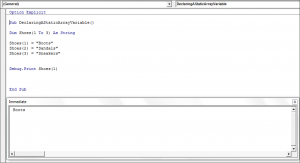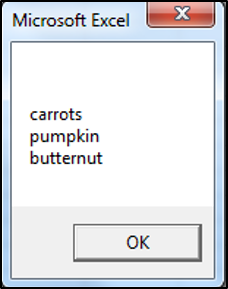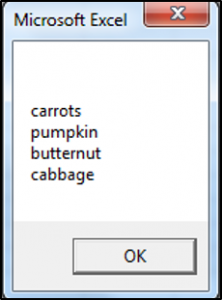### VBA Array Variables

We covered an introduction to variables and constants in our VBA Data Types – Variables and Constants introductory tutorial. In this tutorial, we are going to look at what array variables are and how you can use them in your code.

## What is a VBA Array Variable?

A VBA array variable stores a list or group of elements. A VBA array variable can also be thought of as a group of variables, stored under the same name and having the same data type. All the elements in the array must be the same type. An example would be a list of fruits. An array can store text or numbers. You refer to an element in an array using its index number. You can declare an array variable using the Dim, Static, Public or Private keyword.

### Static Array Variable

A Static array variable has a fixed size or set number of items in the list. You would declare a Static array variable in the following way:

You can display a certain element in the array by referring to its index number as shown in the code below:

If you press F5 to run your code you would get the following result in the Immediate Window:### Dynamic Array Variable

The size of a Dynamic array variable changes at runtime and is set in other words at runtime only. You don’t initially declare the number of items or elements in the array as for the Static array variable, but you use the ReDim keyword to specify the size or the number of items that a Dynamic Array has. You can then resize your array using the ReDim keyword:

When you run this code, you get the message box with all the items in the array that was specified using the ReDim keyword, then you get another message box showing the updated number of items in the array.

The result is:#### You may also like some of this related content...

We covered arrays, static arrays and dynamic arrays in a previous tutorial.  We are going
A VBA Static variable retains its value even when the procedure ends. Declaring a Static
We have already covered an introduction to variables, constants and VBA data types in an
We are going to cover Global Variables, in this tutorial. A variable in VBA can
We have already gone over what variables and constants are, in our VBA Data Types